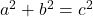Question

The floor of a rectangular room measures 5m by 4m and the ceiling is 3m from the floor. An ant is at the top of a corner of the room and crawls to the opposite bottom corner of the room. Find the shortest distance it can travel. (Cannot do its diagonal distance)

1.thanhcong
3 + √(41) = 9.4 m (nearest tenth)
Step-by-step explanation:
The room can be modeled as a rectangular prism with:
• width = 4 m
• length = 5 m
• height = 3 m
If the ant is at the top of a corner of a room and crawls to the opposite bottom corner of the room, the shortest distance will be to travel down one vertical edge of the room then to travel the diagonal of the floor of the room (or to travel the diagonal of the ceiling and then one vertical edge).
Vertical edge = height of room = 3m
The diagonal of the floor (or ceiling) is the hypotenuse of a right triangle with legs of the width and length.  Therefore, to find the diagonal, use Pythagoras Theorem.
Pythagoras Theoremwhere:
• a and b are the legs of the right triangle
• c is the hypotenuse (longest side) of the right triangle
Given:
• a = width = 4 m
• b = length = 5 m
• c = diagonal
Substitute the given values into the formula and solve for c:Therefore, the shortest distance the ant can travel is:
⇒ 3 + √(41) = 9.4 m (nearest tenth)2.